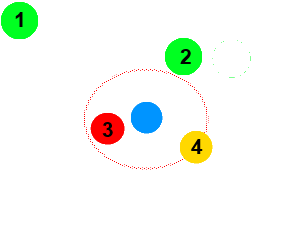## Modeling Herding Behaviors

This article will describe how primsoup implements a herding behavior.

## Concept

After implementing a simple herbivore creature in primsoup, I wanted to give them more interesting behaviors. When I think of large herbivores, I imagine them moving in a herd.

Creating a simulated creature that moved in a herd seemed like an interesting problem. I did not want to make it complicated enough to accurately model a flock of birds. I was aiming for a general "animals stay near - but not on top of - each other" kind of herding.

## To the Videotape!

Sometimes the best way to explain is to show. Here is a video final herding behavior logic at work.

## United We Stand

The key to a herding behavior is wanting to be near other entities. The herding behavior needs to draw an entity toward the entities that it can see. A simple way to do this is to create an attractive force between entities.

But our entities can only move in one direction. That means we need a way to determine a single direction from many sources. A great way to model an attractive force between entities is to copy the gravitational force. That law gives us two useful properties.

• Attraction to nearby entities
• Exponential magnitude### The Reversal!

One problem with gravity is that it is exponential in the wrong direction. If an object is very close, the attractive force is much stronger. If an animal is very close to us in a herd, we are satisfied with that. We want to be powerful attracted to ones that are far away. This makes for a closer herd.

Another problem is gravity only gets stronger as bodies get closer together. However, our herding animals do not want to be TOO close to each other. We need to implement a threshold after which, entities start to repel each other.In the example above, here is how our desired algorithm would quantify the force for each entity. The red border is the desired herd proximity threshold.

1. Strong Attraction
2. Weak Attraction
3. Strong Repulsion
4. No Impact

## Code It

Here is pseudo code for the algorithm we have described.

```				```
float desiredProximity; //set this to the ideal spacing our herd wants
Vector desiredDirection; //our ultimate direction vector
Vector subjectPos; //our subject's current position
List<Entity> entities; //the collection of entities that we are going to consider for our herd
for (Entity e : entities) {

Vector toEntity = e.getPosition().subtract(subjectPos); //calculate the direction from our subject to this entity

float scale = (toEntity.length() - desiredProximity);
bool repulsed = scale < 0;
scale = scale * scale; // square the scale to get exponential magnitude

if(repulsed){
scale *= -1; //we need to reverse the direction if this entity is too close to us
}

Vector scaledDir = toEntity.normalize().scale(scale); //normalize the direction and scale it
desiredDirection = desiredDirection.add(scaledDir); //add this direction into our ultimate desired direction
}
```
```

This algorithm works in 2 and 3 dimensions.

## Going Forward

There are many ways to enhance this algorithm. You could try to implement any of the following:

• Give greater consideration to closer entities - this should cause entities to form smaller pocket herds instead of one larger herd
• Give the creatures some desire to lead the herd - the current algorithm will just blob the entities together. It would be interesting to see entities try to take the lead and move the herd.
• Add some entropy - maybe the herd proximity threshold is not the same (or changes) between entities. What kind of herd would be created by entities who cannot agree on how close the herd should be?